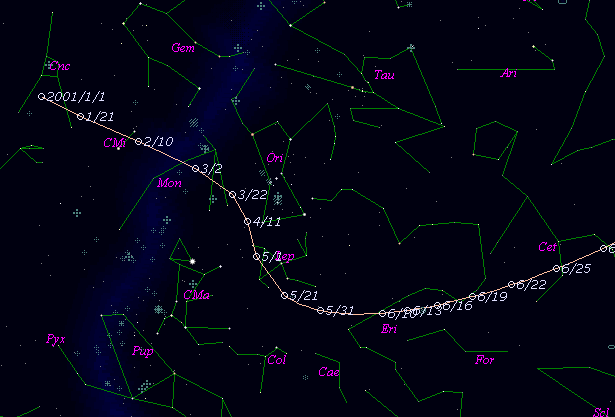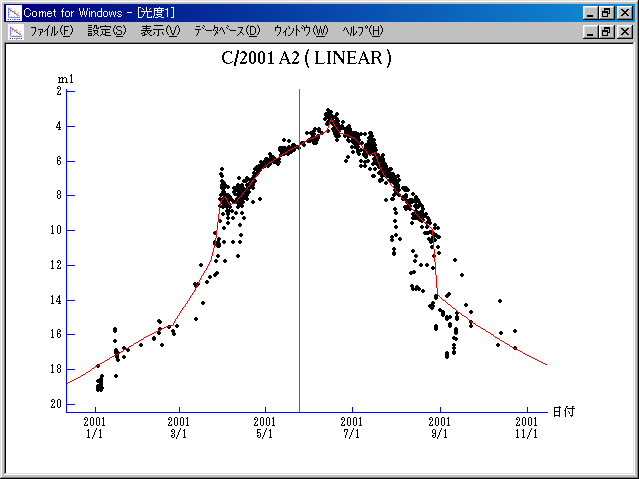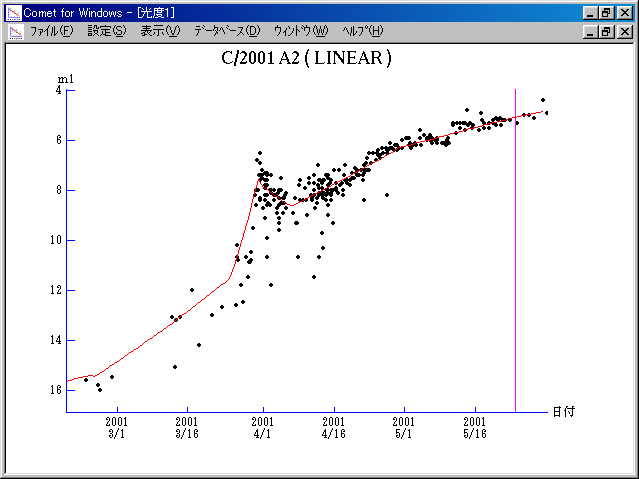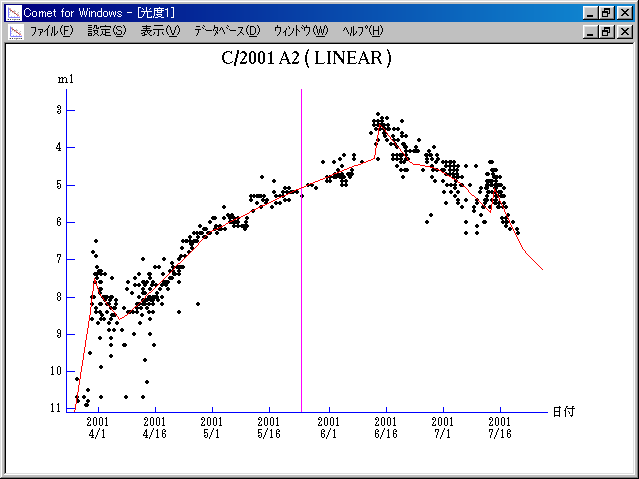# C/2001 A2 ( LINEAR )

 Japanese version Home page Updated on November 25, 2001###Profile

 Discovery Date January 3, 2001 Magnitude 19.0 mag Discoverer Lincoln Laboratory Near-Earth Asteroid Research project

###Orbital Elements

```Epoch 2001 May 11.0 TT = JDT 2452040.5
T 2001 May 24.52041 TT                                  Marsden
q   0.7790265            (2000.0)            P               Q
z  +0.0008544      Peri.  295.32886     -0.47639606     +0.69524253
+/-0.0000023      Node   295.12525     -0.42477634     -0.71796682
e   0.9993344      Incl.   36.47496     -0.76981287     -0.03408035
>From 1381 observations 2001 Jan. 3-Sept. 14, mean residual 0".68.  Non-
gravitational parameters A1 = +0.52 +/- 0.01, A2 = -0.4168 +/- 0.0114.
```

###Finding Charts###Magnitudes Graph

```        m1 = 13.0 + 5 log d +  10.0 log r  [   ,-90]  (             - 2001 Feb. 23)
m1 =  7.0 + 5 log d +  35   log r  [-90,-60]  (2001 Feb. 23 - 2001 Mar. 25)
m1 = -8.0 + 5 log d + 150   log r  [-60,-54]  (2001 Mar. 25 - 2001 Mar. 31)
m1 = 11.0 + 5 log d -  30   log r  [-54,-48]  (2001 Mar. 31 - 2001 Apr.  6)
m1 =  7.5 + 5 log d +  18.0 log r  [-48,-25]  (2001 Apr.  6 - 2001 Apr. 29)
m1 =  7.0 + 5 log d +   6.2 log r  [-25, 20]  (2001 Apr. 29 - 2001 June 13)
m1 =  8.1 + 5 log d +  40   log r  [ 20, 29]  (2001 June 13 - 2001 June 22)
m1 =  7.5 + 5 log d +  10.0 log r  [ 29, 50]  (2001 June 22 - 2001 July 13)
m1 =  4.5 + 5 log d +  35   log r  [ 50, 58]  (2001 July 13 - 2001 July 21)
m1 =  7.5 + 5 log d +  10.0 log r  [ 58, 95]  (2001 July 21 - 2001 Aug. 27)
m1 = 11.0 + 5 log d +  10.0 log r  [ 95,   ]  (2001 Aug. 27 -             )
```##### The orbital elements are calculated by Dr. Brian G. Marsden and printed on M.P.E.C. 2001-R57. The charts are made with StellaNavigator Ver.2.0 for Windows (AstroArts / ASCII). The magnitudes graphs are made with Comet for Windows.